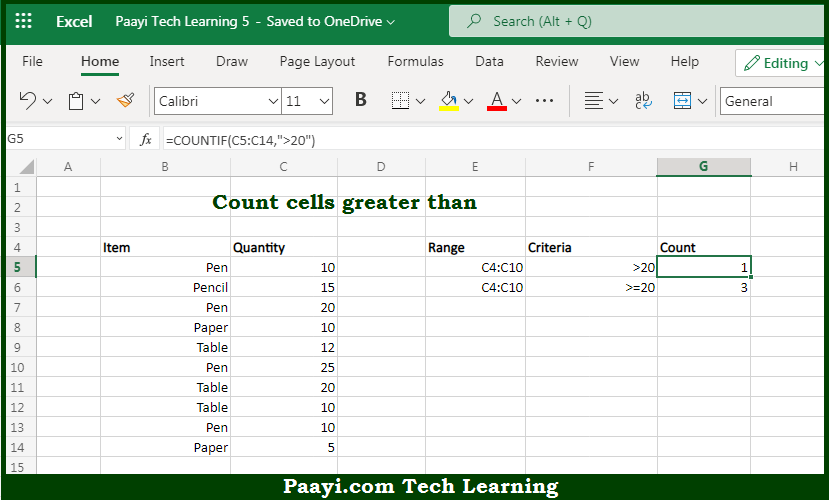# Learn How to Count Cells Greater Than in Microsoft Excel

Written by | 0 Comments | 609 Views

In this article, you will learn how to use the Microsoft Excel COUNT formula and its purpose in Microsoft Excel. You will also get to know how to count cells greater than other cells and see the generic formula.

Count Cells Greater than Using Microsoft Excel Count Formula

The main purpose of the Microsoft Excel COUNT formula is to count the number of cells that have values greater than a particular number. Here we will learn how to count cells greater than other cells.  That implies, with the help of the COUNT formula you can able to count the number of cells that have values greater than a particular number, you can use the COUNTIF function. The generic form of the formula provided below, rng represents a range of cells that contain numbers, and X represents the threshold above which you want to count. So, with the help of the COUNT formula, you can able to count the number of cells that have values greater than a particular number.

General Syntax of the COUNT Cells Count Cells Greater than Others

=COUNTIF(rng,">X")

The Explanation for the Count Cells Greater than OthersSo we know that Microsoft Excel COUNT formula you can able to count the number of cells that have values greater than a particular number. Here we will learn how to count cells greater than other cells. You can use the COUNTIF function to count the number of cells that have values greater than a particular number. In the generic form of the formula, rng represents a range of cells that contain numbers, and X represents the threshold above which you want to count.

It should be noted that COUNTIF counts the number of cells in the range that contain numeric values greater than x, and the result will be number. So, with the help of the COUNT formula, you can able to count the number of cells equal to one of many values.